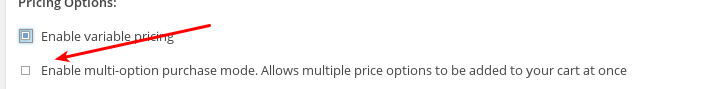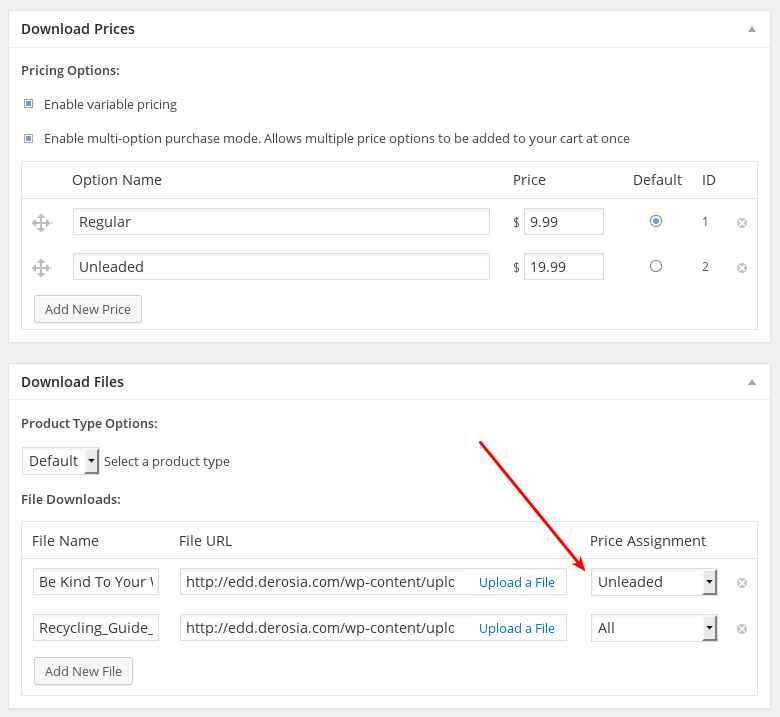• \$ID = 0;
• \$post_author = 0;
• \$post_date = '0000-00-00 00:00:00';
• \$post_date_gmt = '0000-00-00 00:00:00';
• \$post_content = '';
• \$post_title = '';
• \$post_excerpt = '';
• \$post_status = 'publish';
• \$comment_status = 'open';
• \$ping_status = 'open';
• \$post_name = '';
• \$to_ping = '';
• \$pinged = '';
• \$post_modified = '0000-00-00 00:00:00';
• \$post_modified_gmt = '0000-00-00 00:00:00';
• \$post_content_filtered = '';
• \$post_parent = 0;
• \$guid = '';
• \$post_mime_type = '';
• \$comment_count = 0;
• \$filter;

Note: The above properties are inherited from and identical to the properties in WP_Post.

To create a new download you first instantiate the class and then run the create() method.

```\$new_download = new EDD_Download;
```

The create method uses  wp_insert_post(), so you may pass any arguments to create() that wp_insert_post() can accept.  Something like this would work fine:

```\$new_download = new EDD_Download;

'post_title'    => 'My eBook',
'post_content'  => 'This is my eBook. Nice long description.',
'post_status'   => 'publish',
)
```

This is accomplished simply by passing a Download ID to the class name during instantiation.

Example:

```\$my_download = new EDD_Download( 1492 );
```

### Data Fetching Methods

##### get_ID()

Example:

```\$download_id = \$my_download->get_ID();
```

Returns: an integer, like 1492.

##### get_price()

This method returns the price of the Download in double format, with no currency symbol.

Example:
```\$download_price = \$my_download->get_price();
```

Returns: a double, like 9.99. Math may be done on this result.

##### get_prices()

This method returns an array of the variable prices of the Download in double format, with no currency symbol.

Example:
```\$variable_prices = \$my_download->get_prices();
```
Returns an array of prices like this:
```Array
(
 => Array
(
[index] => 1
[name] => Regular
[amount] => 9.99
)

 => Array
(
[index] =>
[amount] => 19.99
)
```
##### is_single_price_mode()

Determines whether Single Price Mode is enabled or disabled.  Single price mode refers to whether multiple price options can be purchased simultaneously.  In the Easy Digital Downloads user interface the checkbox for it is under Variable Pricing.Example:
```\$is_single_price_mode = \$my_download->is_single_price_mode();
```

Returns: true or false

##### has_variable_prices()

Determines whether Variable Pricing is enabled or disabled on a specific Download.

Example:
```\$download_price = \$my_download->has_variable_prices();
```

Returns: true or false

##### get_files()

This method returns an array of the files attached to the Download

Example:
```\$my_files = \$my_download->get_files();
```
Returns an array of prices like this:
```Array
(
 => Array
(
[attachment_id] => 10
[name] => Be Kind To Your Web Footed Friends
[condition] => all
)

)
```

This method gets the number of times this file may be downloaded.

Example:

```\$download_limit = \$my_download->get_file_download_limit();
```

Returns: an integer, like 100.

##### get_file_price_condition()

When a product has variable pricing, one or more files may available for purchase. Under the Price Assignment a File Download can be associated with All price options, or only specific ones.  In the example below the file is associated with the Unleaded price option.Example:

```\$price_condition = \$my_download->get_file_price_condition();
```

Returns: an integer, like 2.

##### get_type()

Example:

```\$download_type = \$my_download->get_type();
```

Returns: either 'default' or 'bundle'

Example:

```\$is_bundled_download = \$my_download->is_bundled_download();
```

Returns: true or false

Example:

```\$bundled_downloads = \$my_download->get_bundled_downloads();
```

```Array
(
 => 68
 => 114
)
```

Note: get_bundled_downloads() will return an empty array if the product is not a bundle, so look at doing something like this:

```if ( \$my_download->is_bundled_download() ) {
}
```
##### get_notes()

Example:

```\$download_notes = \$my_download->get_notes();
```

Returns: The notes as a string, and maintains line breaks.

##### get_sku()

Example:

```\$download_sku = \$my_download->get_sku();
```

Returns: The sku as a string

##### get_button_behavior()

Returns the what happens when someone clicks the buy button

Example:

```\$download_button_behavior = \$my_download->get_button_behavior();
```

##### get_sales()

Returns the number of sales completed for this item

Example:

```\$download_sales = \$my_download->get_sales();
```

Returns: an integer like 42

##### increase_sales()

Increments the number of sales by 1. Cannot take input, cannot increase by more than 1.

Example:

```\$increase_sales = \$my_download->increase_sales();
```

Returns: either false on failure or an integer of the new sales count.

##### decrease_sales()

Decrements the number of sales by 1. Cannot take input, cannot increase by more than 1.

Example:

```\$decrease_sales = \$my_download->decrease_sales();
```

Returns: either false on failure or an integer of the new sales count.

##### get_earnings()

Example:

```\$download_earnings = \$my_download->get_earnings();
```

Returns: a float like 152.69

##### increase_earnings( mixed \$amount = 0 )

Example:

```\$increase_earnings = \$my_download->increase_earnings();
```

Returns: either false on failure or a float of the new amount, like 247.39

##### decrease_earnings( mixed \$amount = 0 )

Example:

```\$decrease_earnings = \$my_download->decrease_earnings();
```

Returns: either false on failure or a float of the new amount, like 147.39

##### is_free( mixed \$price_id = false )

Checks to see if the download is free, OR if the given price ID is free.

Example:

```\$is_free = \$my_download->is_free();
```

Returns: true or false